### Geometry II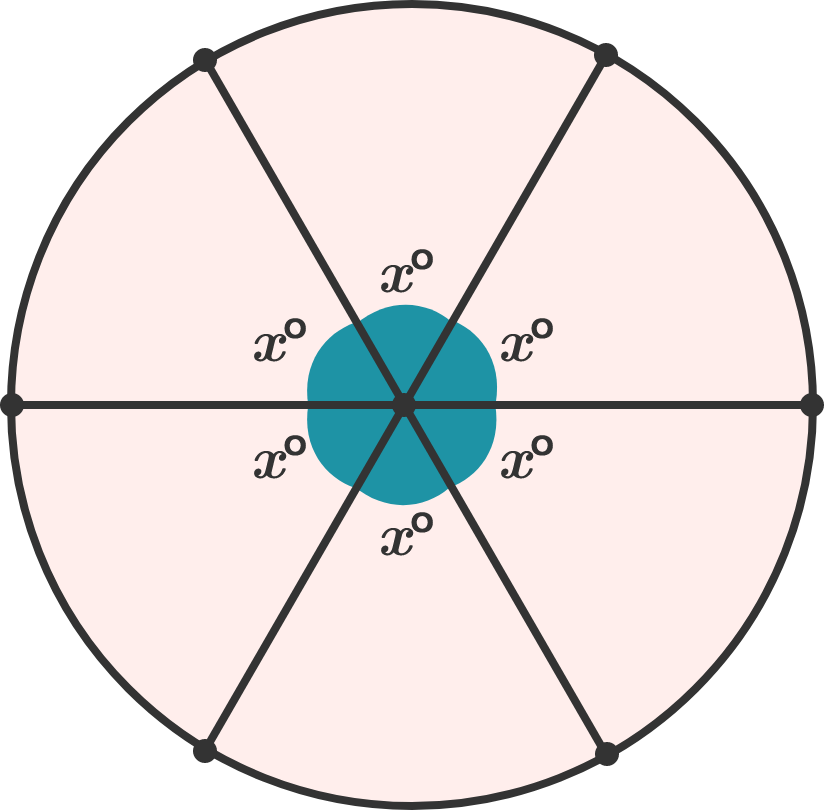If three diameters on a circle make six congruent angles as shown, what is $x?$

# Central Angles and Arcs

Welcome to Intermediate 2D Geometry! This course follows Geometry I.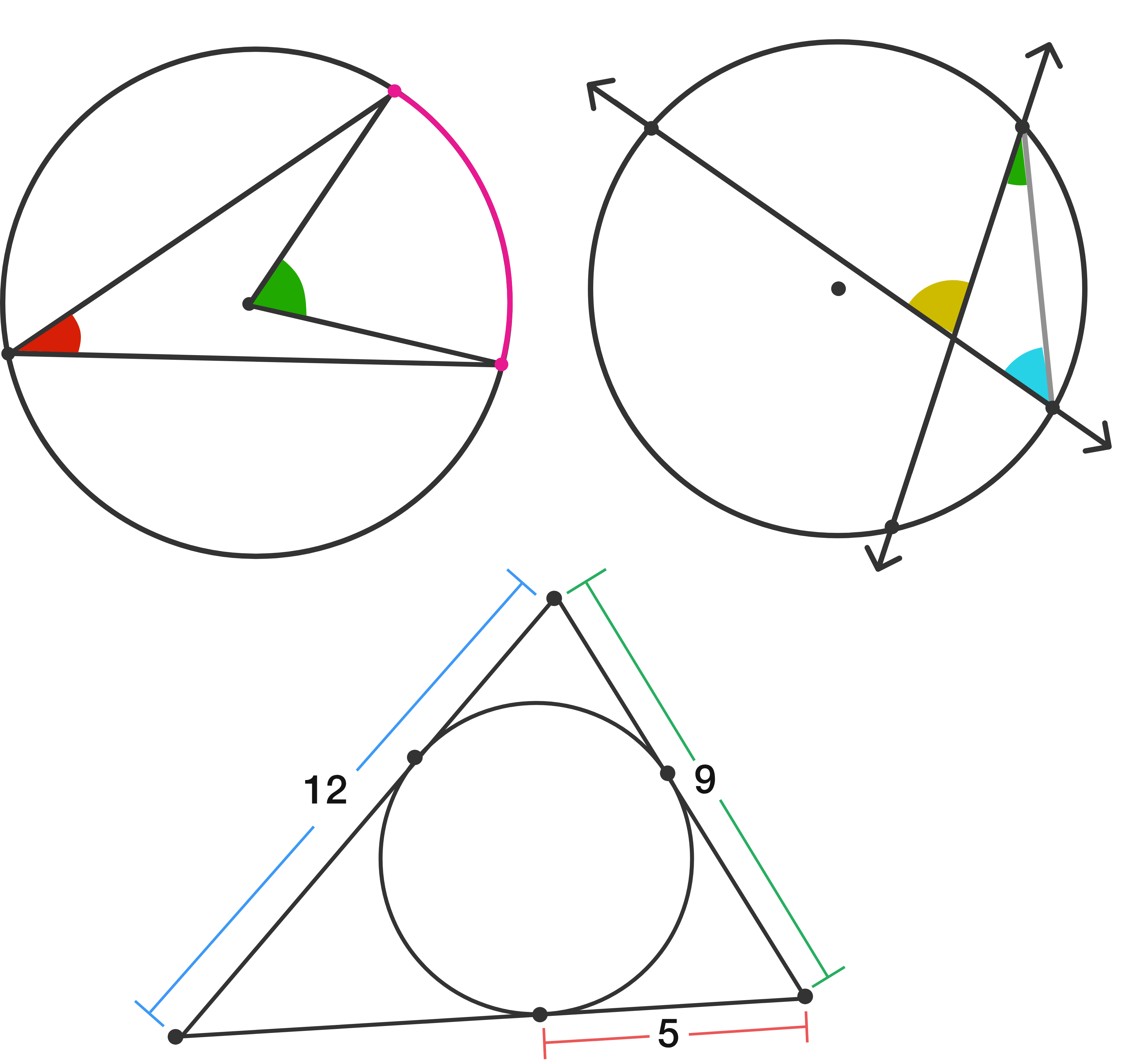The focus of the course is on circles, triangles, and triangle centers, all of which are interrelated. For now, though, let's stick with the humble circle.

# Central Angles and Arcs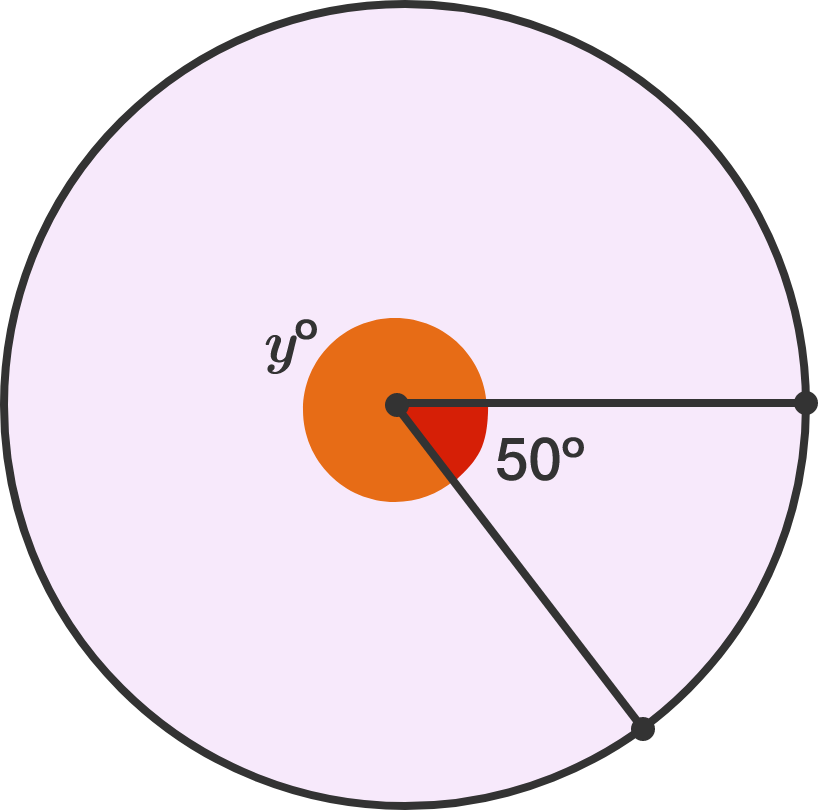A central angle is one where the vertex of the angle is on the center of a circle. $50^\circ$ in the diagram is a central angle; what is $y ?$

# Central Angles and Arcs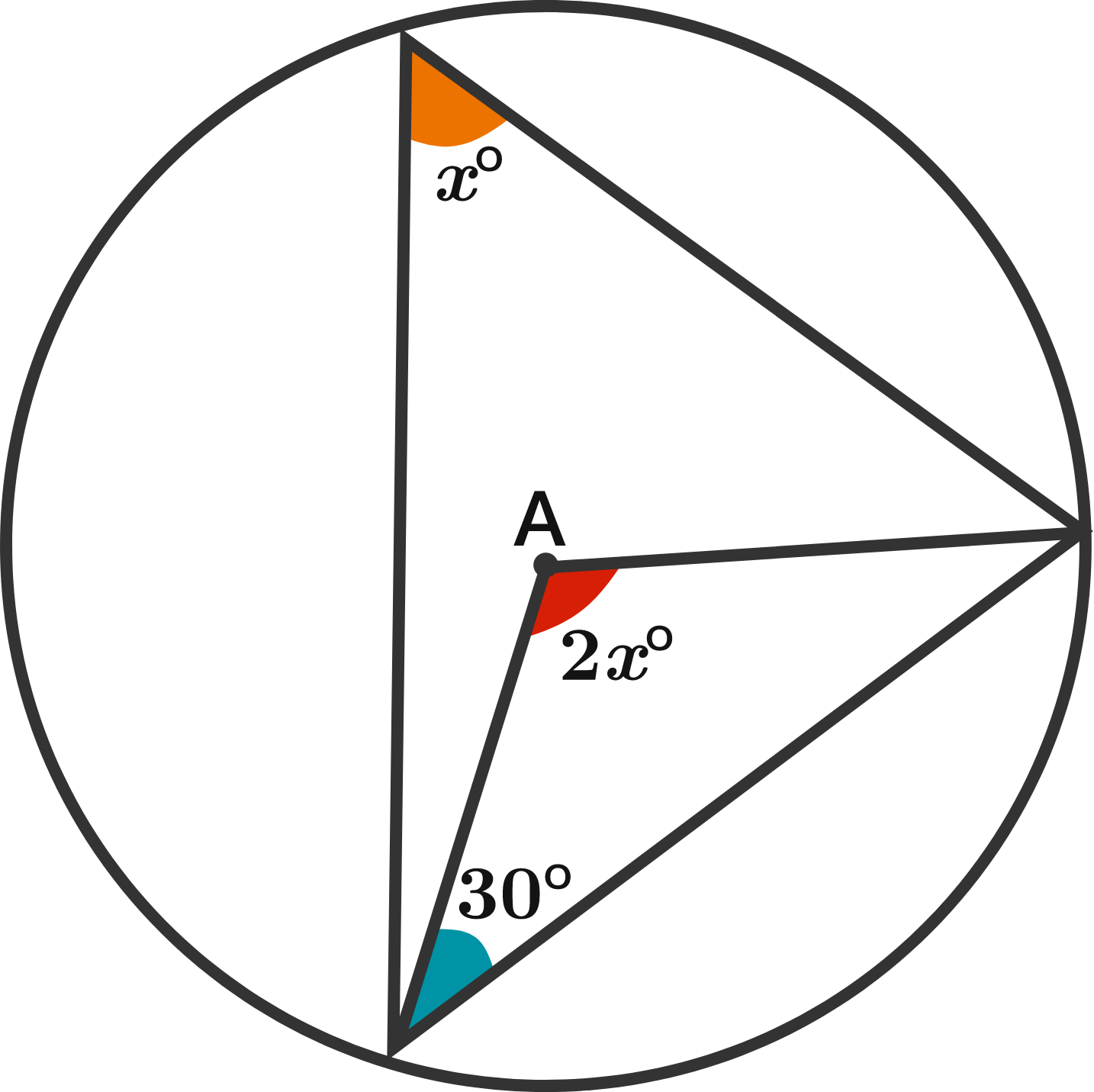A is the center of a circle; all other points on the triangles touch the circle. Solve for $x .$

# Central Angles and Arcs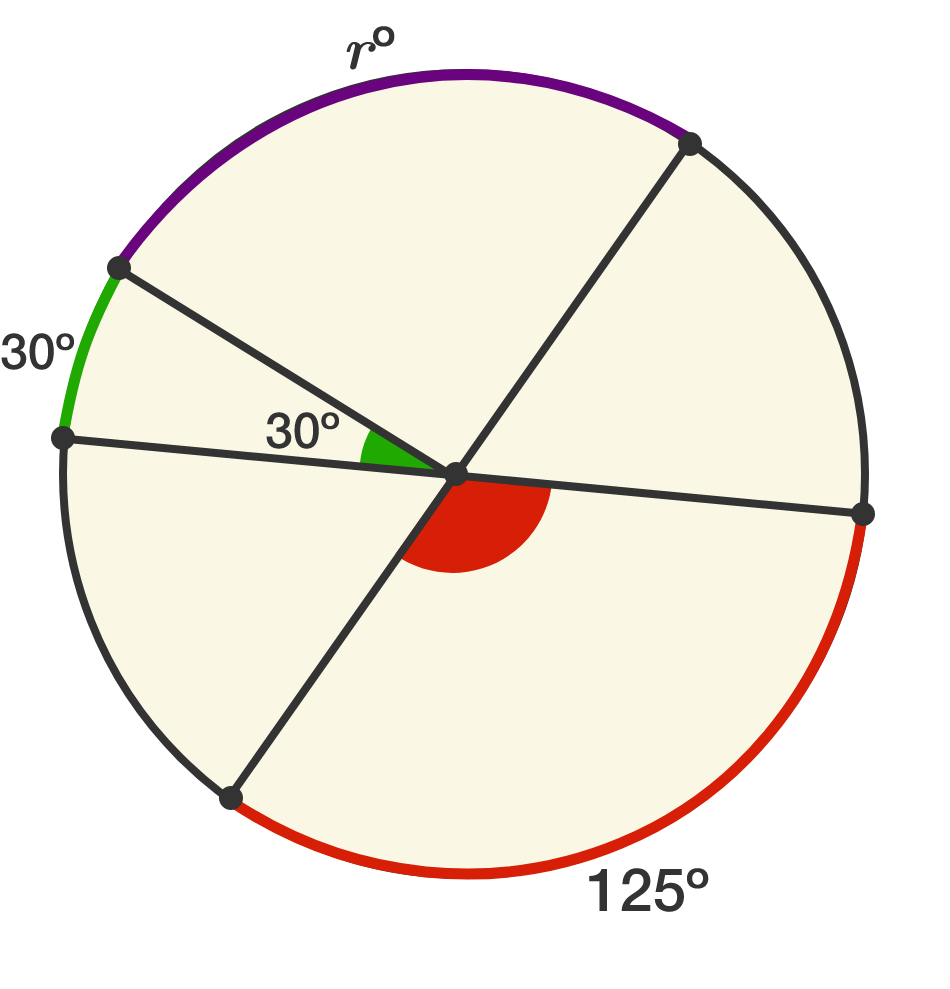Arcs of circles have measures just like angles; their measure is identical to the central angle that subtends them (that is, the central angle that touches the endpoints of the arc).

The diagram above is drawn with two diameters and one radius; if $r^\circ$ is the measure of the purple arc, what is $r?$

# Central Angles and Arcs

The short red arc below is notated either $\overparen{AE}$ or $\overparen{EA} .$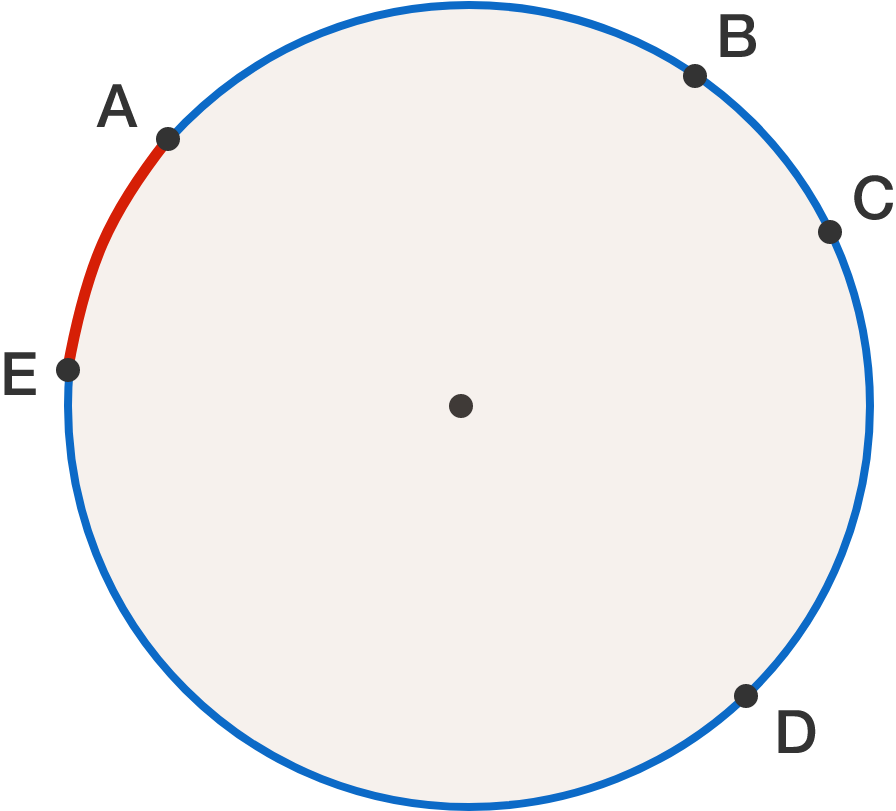At least some letters need to be added (in the order they appear) to indicate the longer blue arc. For example,

$\overparen{ABE} \quad \overparen{ABCDE} \quad \overparen{ECBA}$

all work. Including the three ways above, how many ways are there to denote the blue arc?

Note that "out of order" listing between the points isn't allowed, like $\overparen{ADCBE} .$

# Central Angles and Arcs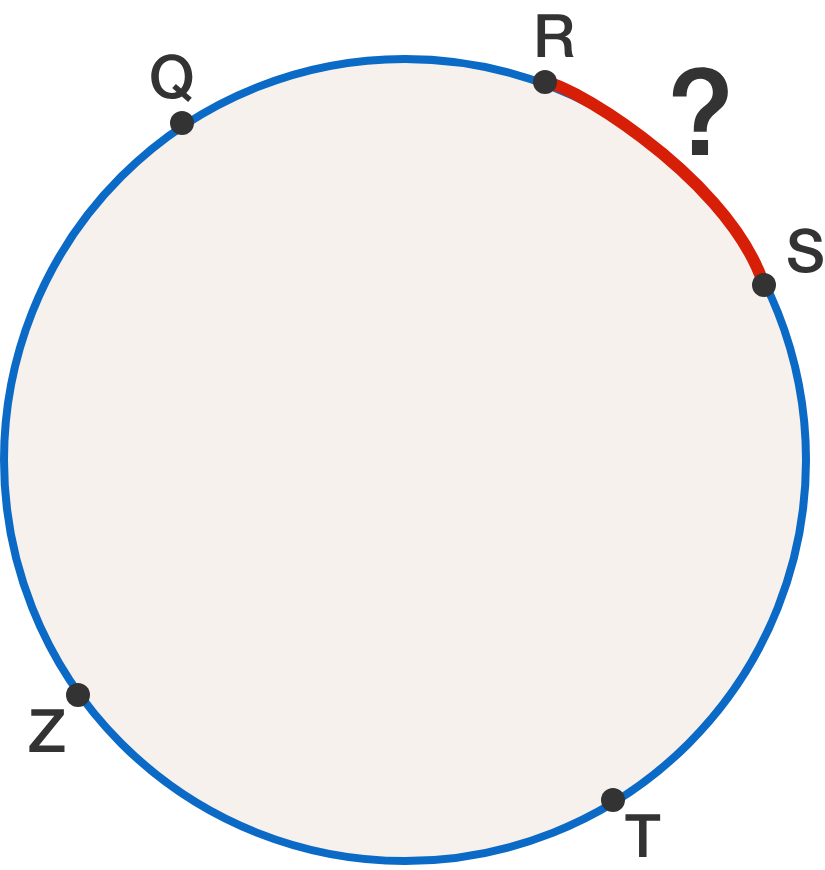$\overparen{QZT}$ is a semicircle, $\overparen{QRS} = 100^\circ ,$ and $\overparen{RST} = 120^\circ .$ What is the measure of arc $\overparen{RS} ?$

×